# Roku SDK Documentation : ifSGNodeBoundingRect

## Introduction

Available since firmware version 7.0

The ifSGNodeBoundingRect interface can be used to query the bounding rectangle of subject node. The ifSGNodeBoundingRect interface methods return a node bounding rectangle as an associative array with four elements:

NameValue
xx-coordinate of the origin of the bounding rectangle
yy-coordinate of the origin of the bounding rectangle
widthwidth of the bounding rectangle
heightheight of the bounding rectangle
These methods return the bounding rectangle dimensions and location of component objects at the time they are called. If they are called before an object is fully constructed, such as before all graphical images have been loaded, they will return the dimensions and location at the time of the call, which may not be the correct values for placing the component object properly. To ensure that your screen has the component objects located as you intended, make sure you call these methods after the component object is fully constructed. For example, if the component object relies on loading graphical images to construct its appearance, it is best to use these methods as part of an observer callback function triggered by the image loading field events, such as the `loadStatus` field of the Poster node.

## Description of Methods

MethodDescription

#### localBoundingRect()

`localBoundingRect() as Object`

Returns the node local bounding rectangle as an associative array. The local bounding rectangle of a node is the axis-aligned rectangle, that includes the union of the bounding rectangle of the geometry of the node, and the bounding rectangles of all of the node children, transformed into the local coordinate system of the node.

#### boundingRect()

`boundingRect() as Object`

Returns the node bounding rectangle as an associative array. The bounding rectangle of a node is the axis-aligned rectangle computed by transforming the local bounding rectangle of the node by the node transformation matrix. The resulting rectangle corresponds to the node local bounding rectangle transformed into its parent node local coordinate system.

#### sceneBoundingRect()

`sceneBoundingRect() as Object`

Returns the bounding rectangle as an associative array for scene components (component nodes extended from a Scene or OverhangPanelSetScene node class). If `sceneBoundingRect()` is called before any child components have been added to the Scene component, or the child components are smaller than the Scene component, this is the screen dimensions of the user interface, so can be used to automatically create a bounding rectangle for the specified display user interface dimensions (SD, HD, FHD), to locate and dimension child components within the screen. If `sceneBoundingRect()` is called after child components have been added that are larger than the Scene component, the returned bounding rectangle will be larger than the user interface dimensions.

#### ancestorBoundingRect()

`ancestorBoundingRect(ancestornode as Object) as Object`

Returns the bounding rectangle as an associative array for the specified `ancestornode`, which can be any node in the tree above the calling node up to the Scene node.

#### localSubBoundingRect()

`localSubBoundingRect(itemnumber as String) as Object`

#### subBoundingRect()

`subBoundingRect(itemnumber as String) as Object`

#### sceneSubBoundingRect()

`sceneSubBoundingRect(itemnumber as String) as Object`

#### ancestorSubBoundingRect()

`ancestorSubBoundingRect(itemnumber as String) as Object`

These methods return the bounding rectangle as an associative array for an item in a grid node (a grid node class derived from ArrayGrid) specified by `itemnumber`, where `number` is the index number of the grid item (see Creating Lists and Grids). As an example, for a grid node `grid`, the following will return the Scene node bounding rectangle `rect` of the grid item index number 12:

`rect = grid.sceneSubBoundingRect("item12")`

## Sample Channel Illustrating Bounding Rectangles

The scene has the following parenting structure:

Scene
RectangleGroup
Rectangle (Green)
Rectangle (Yellow)

```<Scene>
<Group id="RectGroup" translation="[100, 50]" >
<Rectangle id="GreenRect" width="100" height="150" color="0x00FF00FF" translation="[200, 75]" >
<Rectangle id="YellowRect" width="150" height="250" color="0xFFFF00FF" translation="[200,100]" rotation="-0.45"/>
</Rectangle>
</Group>
</Scene>```

Initially, the illustration shows the local coordinates systems of each of these nodes.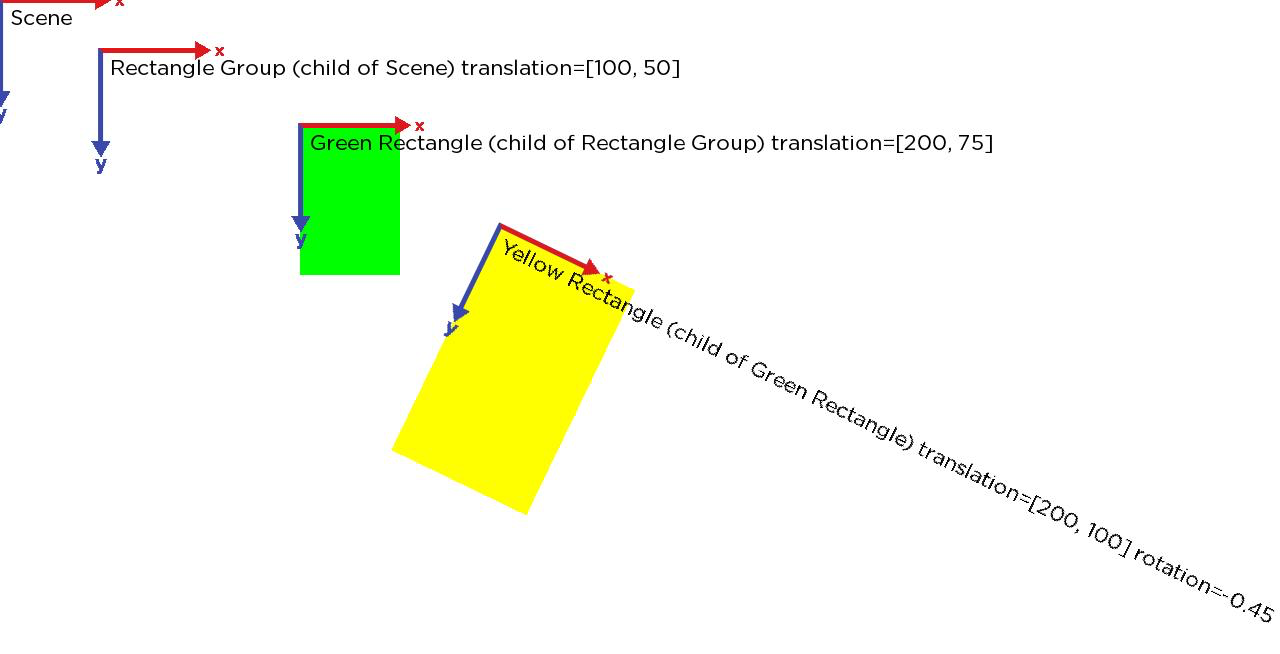The next illustration shows the Yellow rectangle's localBoundingRect().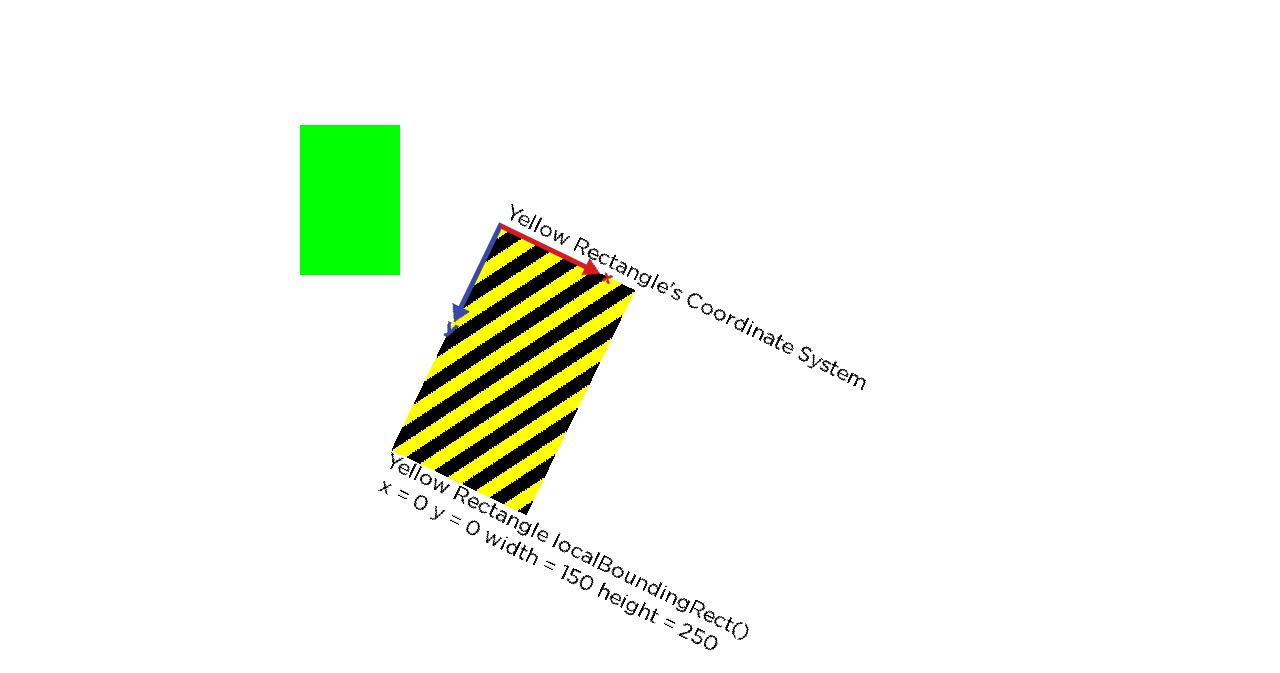The next illustration shows the Yellow rectangle's boundingRect(), which is relative to it's parent's coordinate system.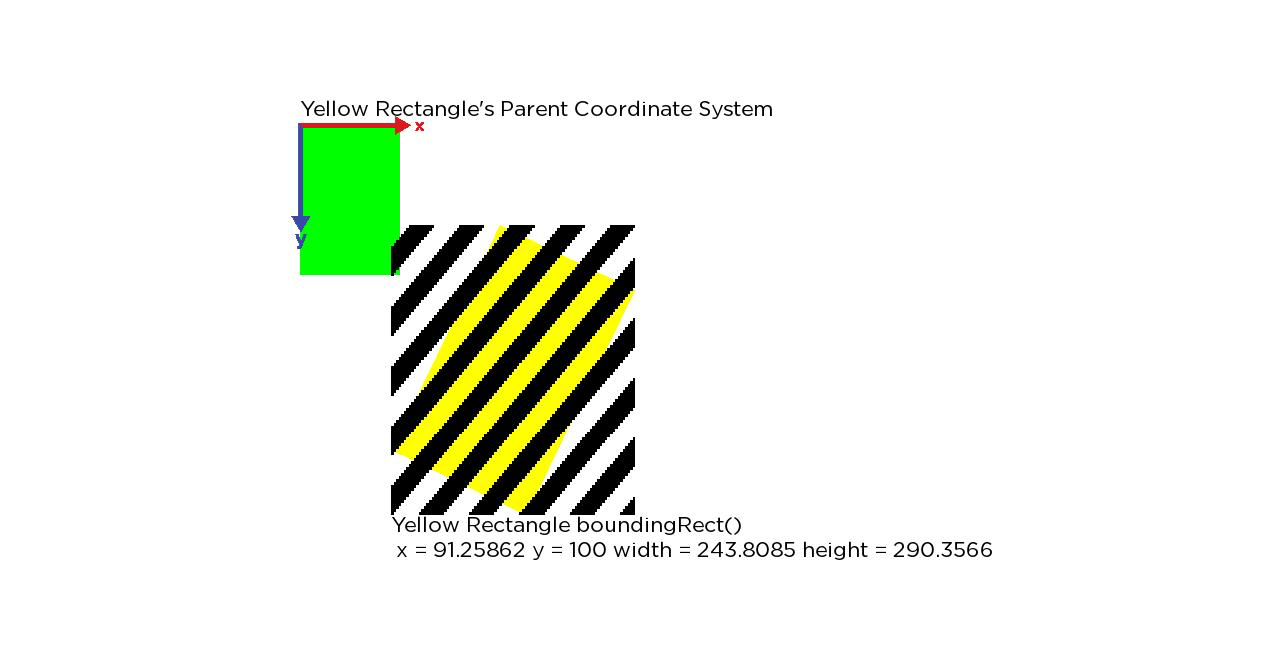The next illustration shows the Yellow rectangle's sceneBoundingRect(), which is relative to the Scene's coordinate system.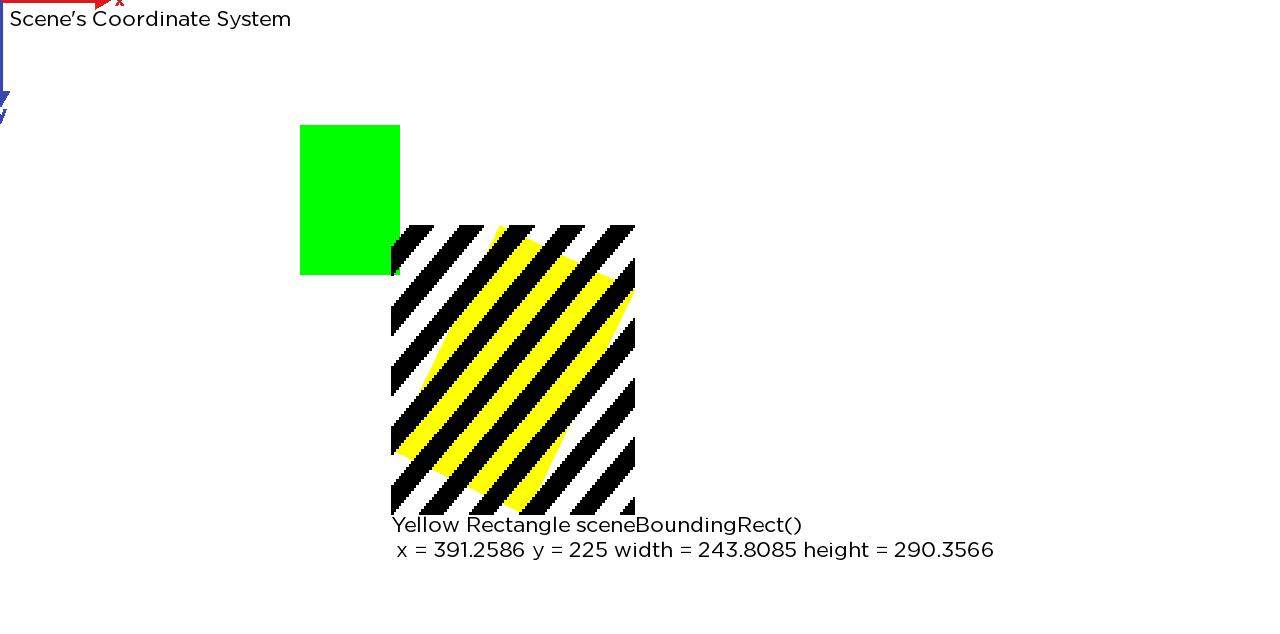The next illustration shows the localBoundingRect() of the Green rectangle, that includes the union of the Green rectangle's local rectangle and the boundingRect() of it's child. This rectangle is relative to the Green rectangle's local coordinate system.

Note that this is the smallest, screen-aligned rectangle the encloses both the Green and Yellow rectangle.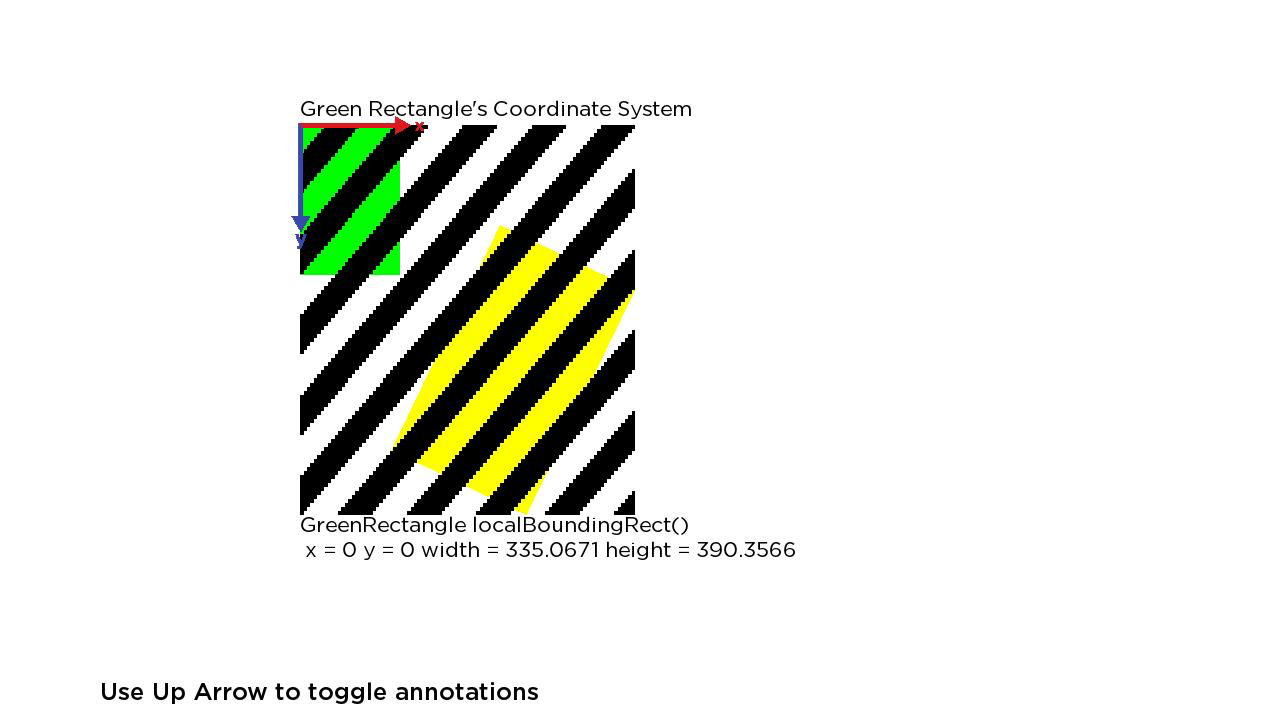The next illustration shows the boundingRect() of the Green rectangle, which is relative to its parent Group's coordinate system.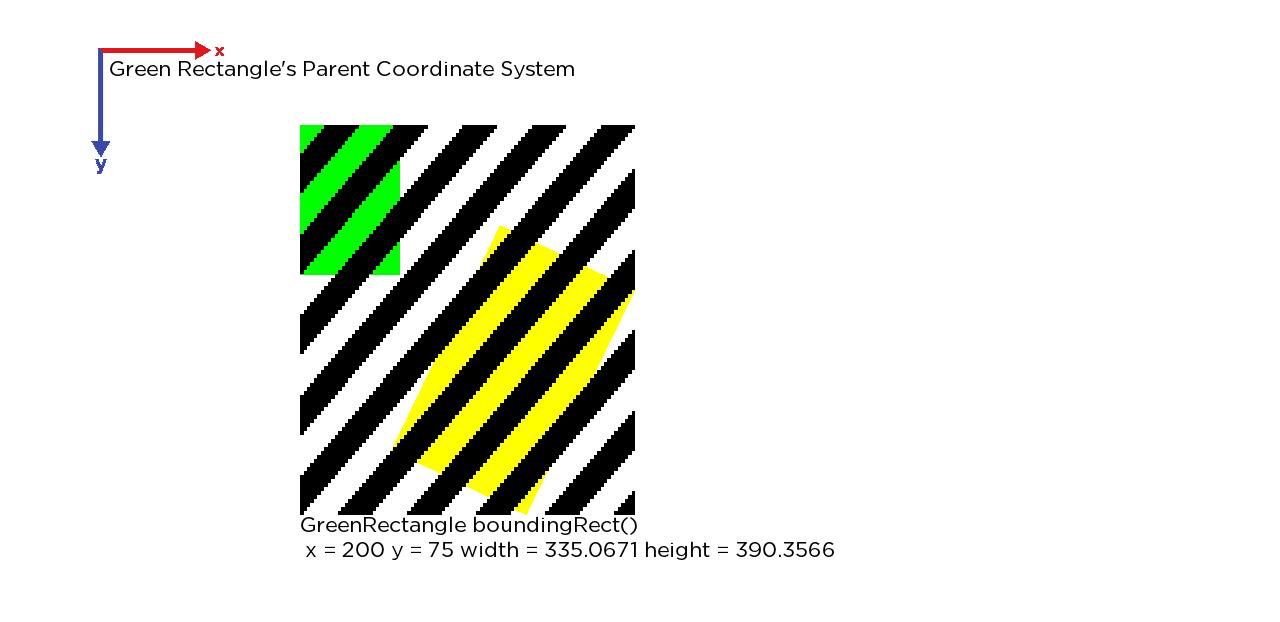The last illustration shows the Green rectangle's sceneBoundingRect(), which is relative to the Scene's coordinate system.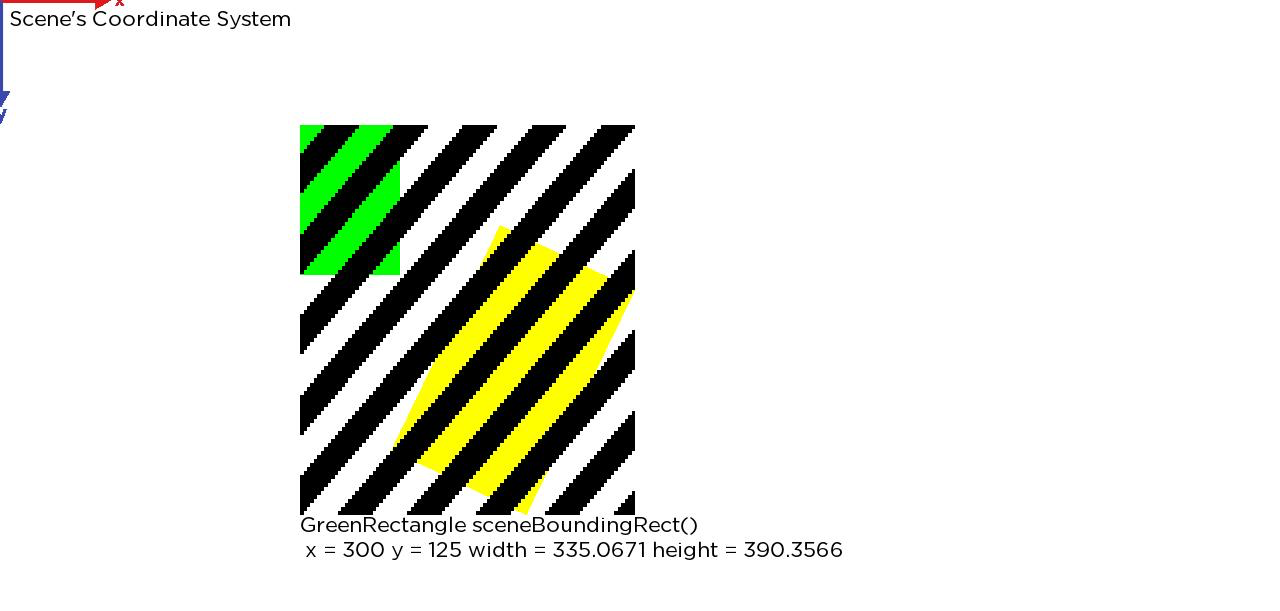## Attachments: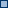rectangle-group1.jpg (image/jpeg)rectangle-group1.png (image/png)rectangle-group2.png (image/png)rectangle-group3.png (image/png)rectangle-group4.png (image/png)rectangle-group5.png (image/png)rectangle-group6.png (image/png)rectangle-group7.png (image/png)boundingRecttest.zip (application/zip)